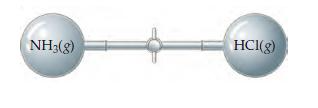Problem: Ammonia, NH3(g), and hydrogen chloride, HCl(g), react to form solid ammonium chloride, NH4Cl(s):NH3(g) + HCl(g) → NH4Cl(s)Two 2.50 L flasks at 30.0 oC are connected by a stopcock, as shown in the drawingOne flask contains 5.60g NH3(g), and the other contains 4.60 g  HCl(g). When the stopcock is opened, the gases react until one is completely consumed.What will be the final pressure of the system after the reaction is complete? (Neglect the volume of the ammonium chloride formed.)

FREE Expert Solution
81% (133 ratings)
FREE Expert Solution

Balanced reaction: NH3(g) + HCl(g) → NH4Cl(s)

Step 1: Calculate the number of moles of each reactant then determine the amount of products produced by each

Molar masses of  NH3(g) and HCl(g) will be needed to convert grams to moles

81% (133 ratings)
Problem Details

Ammonia, NH3(g), and hydrogen chloride, HCl(g), react to form solid ammonium chloride, NH4Cl(s):
NH3(g) + HCl(g) → NH4Cl(s)
Two 2.50 L flasks at 30.0 oC are connected by a stopcock, as shown in the drawingOne flask contains 5.60g NH3(g), and the other contains 4.60 g  HCl(g). When the stopcock is opened, the gases react until one is completely consumed.

What will be the final pressure of the system after the reaction is complete? (Neglect the volume of the ammonium chloride formed.)